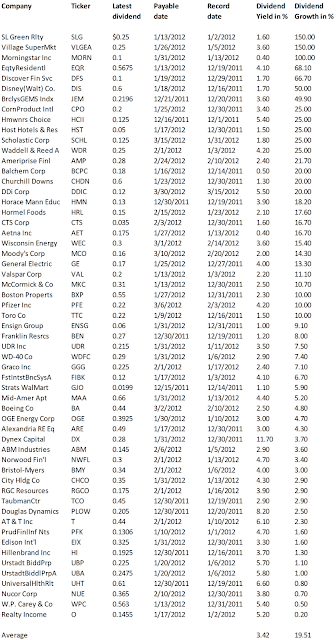## Pages

### Stocks With Fastest Dividend Growth December 2011

Shares With Highest Dividend Growth by Dividend Yield – Stock, Capital, Investment. Here is a current sheet of companies with fastest dividend growth compared to the previous dividend declaration. The dividend growth is often a good indicator for the financial health of a stock. Companies with a strong increase in dividends judge the future of their company rosy. They give money back to shareholders that they don’t need for their business. In total, 57 stocks increased dividends of which 28 raised dividends by more than 10 percent.

Here are the 5 top stocks with fastest dividend growth by dividend yield:

Dynex Capital, Inc. (NYSE:DX) has a market capitalization of \$377.16 million. The company employs 15 people, generates revenues of \$48.78 million and has a net income of \$29.47 million. The firm’s earnings before interest, taxes, depreciation and amortization (EBITDA) amounts to \$24.52 million. Because of these figures, the EBITDA margin is 50.27 percent (operating margin 56.20 percent and the net profit margin finally 60.42 percent).

The total debt representing 81.31 percent of the company’s assets and the total debt in relation to the equity amounts to 458.78 percent. Due to the financial situation, the return on equity amounts to 12.60 percent. Finally, earnings per share amounts to \$1.08 of which \$0.98 were paid in form of dividends to shareholders last fiscal.

Here are the price ratios of the company: The P/E ratio is 8.64, Price/Sales 7.72 and Price/Book ratio 0.97. Dividend Yield: 12.02 percent. The beta ratio is 0.45.Long-Term Stock Chart Of Dynex Capital, Inc. (Click to enlarge)

Universal Health Realty Income Trust (NYSE:UHT) has a market capitalization of \$469.88 million. The company generates revenues of \$28.88 million and has a net income of \$16.31 million. The firm’s earnings before interest, taxes, depreciation and amortization (EBITDA) amounts to \$21.33 million. Because of these figures, the EBITDA margin is 73.85 percent (operating margin 46.27 percent and the net profit margin finally 56.48 percent).

The total debt representing 31.26 percent of the company’s assets and the total debt in relation to the equity amounts to 46.46 percent. Due to the financial situation, the return on equity amounts to 11.39 percent. Finally, earnings per share amounts to \$1.21 of which \$2.42 were paid in form of dividends to shareholders last fiscal.

Here are the price ratios of the company: The P/E ratio is 30.61, Price/Sales 16.14 and Price/Book ratio 3.20. Dividend Yield: 6.63 percent. The beta ratio is 0.63.Long-Term Stock Chart Of Universal Health Realt... (Click to enlarge)

Homeowners Choice (NASDAQ:HCII) has a market capitalization of \$49.39 million. The company employs 93 people, generates revenues of \$68.62 million and has a net income of \$5.42 million. The firm’s earnings before interest, taxes, depreciation and amortization (EBITDA) amounts to \$16.07 million. Because of these figures, the EBITDA margin is 23.42 percent (operating margin 12.51 percent and the net profit margin finally 7.90 percent).

The total debt representing 0.00 percent of the company’s assets and the total debt in relation to the equity amounts to 0.00 percent. Due to the financial situation, the return on equity amounts to 11.79 percent. Finally, earnings per share amounts to \$0.98 of which \$0.10 were paid in form of dividends to shareholders last fiscal.

Here are the price ratios of the company: The P/E ratio is 8.18, Price/Sales 0.72 and Price/Book ratio 1.06. Dividend Yield: 6.29 percent.Long-Term Stock Chart Of Homeowners Choice, Inc. (Click to enlarge)

AT&T (NYSE:T) has a market capitalization of \$170.31 billion. The company employs 256,210 people, generates revenues of \$124,280.00 million and has a net income of \$19,400.00 million. The firm’s earnings before interest, taxes, depreciation and amortization (EBITDA) amounts to \$38,952.00 million. Because of these figures, the EBITDA margin is 31.34 percent (operating margin 15.75 percent and the net profit margin finally 15.61 percent).

The total debt representing 24.64 percent of the company’s assets and the total debt in relation to the equity amounts to 59.26 percent. Due to the financial situation, the return on equity amounts to 17.90 percent. Finally, earnings per share amounts to \$1.97 of which \$1.69 were paid in form of dividends to shareholders last fiscal.

Here are the price ratios of the company: The P/E ratio is 14.59, Price/Sales 1.37 and Price/Book ratio 1.53. Dividend Yield: 5.97 percent. The beta ratio is 0.60.Long-Term Stock Chart Of AT&T Inc. (Click to enlarge)

Urstadt Biddle Properties (NYSE:UBA) has a market capitalization of \$500.43 million. The company employs 32 people, generates revenues of \$85.15 million and has a net income of \$27.85 million. The firm’s earnings before interest, taxes, depreciation and amortization (EBITDA) amounts to \$50.35 million. Because of these figures, the EBITDA margin is 59.13 percent (operating margin 41.44 percent and the net profit margin finally 32.71 percent).

The total debt representing 23.30 percent of the company’s assets and the total debt in relation to the equity amounts to 32.16 percent. Due to the financial situation, the return on equity amounts to 6.30 percent. Finally, earnings per share amounts to \$0.65 of which \$0.97 were paid in form of dividends to shareholders last fiscal.

Here are the price ratios of the company: The P/E ratio is 26.13, Price/Sales 5.83 and Price/Book ratio 2.04. Dividend Yield: 5.72 percent. The beta ratio is 0.66.Long-Term Stock Chart Of Urstadt Biddle Propert... (Click to enlarge)

Here is the full list of stocks with fastest dividend growth:Stocks With Fastest Dividend Growth December 2011 (Click to enlarge)

Related Stock Ticker:
SLG, VLGEA, MORN, EQR, DFS, DIS, JEM, CPO, HCII, HST, SCHL, WDR, AMP, BCPC, CHDN, DDIC, HMN, HRL, CTS, AET, WEC, MCO, GE, VAL, MKC, BXP, PFE, TTC, ENSG, BEN, UDR, WDFC, GGG, FIBK, GJO, MAA, BA, OGE, ARE, DX, ABM, NWFL, BMY, CHCO, RGCO, TCO, PLOW, T, PFK, EIX, HI, UBP, UBA,UHT, NUE, WPC, O,

Selected Articles: